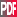11th Conference on Atmospheric Radiation
11th Conference on Cloud Physics

JP4.16

An iterative method for generating scaling log-normal simulations

Gregory M. Lewis, Univ. of British Columbia, Vancouver, BC, Canada; and P. H. Austin

It is has been shown that the single-point probability distributions of certain cloud properties, such as cloud optical depth, are positively skewed and resemble the log-normal distribution. There is also evidence that, for certain spatial scales, the two-point statistics are such that the energy spectrum Ek satisfies a power-law relation, with scale invariant exponent beta lying between 1 and 3, i.e. Ek ~ k^{-beta}, 1< beta < 3, where k is the wave number. Thus, it would be useful to be able to generate scaling log-normal random fields, i.e. simulations with log-normal single-point statistics and energy spectra that satisfy Ek ~ k^{-beta}. Evans et al. (1999) have presented a method that can generate such simulations when beta<1. Here we describe and test a method that is also valid for 1 < beta< 3.

The Gaussian distribution is a stable distribution, and so, a Gaussian random field with any desired energy spectrum may be generated quite easily using Fourier-space filtering. A log-normal simulation may then be generated by exponentiating this Gaussian random field. However, the difficulty arises because a log-normal field generated in this manner will not have the same energy spectrum as the original Gaussian field. Ideally, it would be possible to analytically determine the energy spectrum for the Gaussian field, such that exponentiation of this field results in a log-normal field with the desired energy spectrum. This approach is implemented in the method of Evans et al. (1999), which is valid for the case beta<1.

We extend the range of validity to 1 < beta < 3 by using a simple iterative method to numerically determine the appropriate energy spectrum for the Gaussian field. The iteration produces a sequence of Gaussian random fields, where the difference between the desired energy spectrum (for the log-normal field) and the energy spectrum of the exponentiation of a field in the sequence is used to generate the energy spectrum for the next Gaussian field in the sequence (i.e. the next iterate). The main assumption of the method is that the exponentiation of the Gaussian field does not substantially alter the form of the energy spectrum. This is a valid assumption when the mean and the variance of the Gaussian field are not large, which is the case of interest for cloud statistics. We present results from this technique and discuss its performance, including the variance range for which convergence occurs.Extended Abstract (140K)